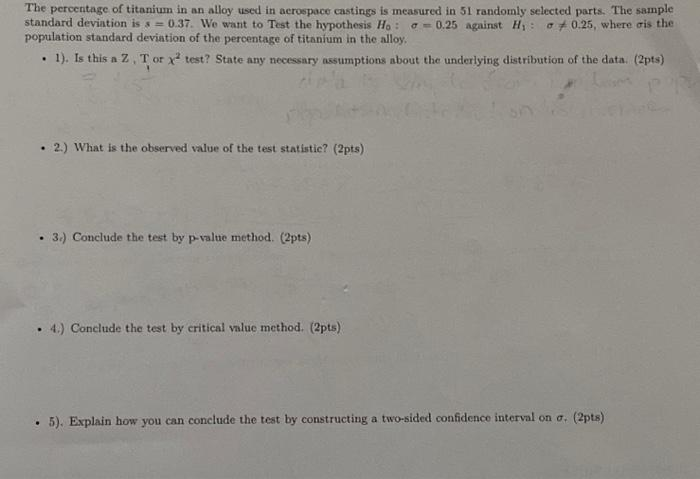# (Solved): please answer and show your work The percentage of titanium in an alloy used in aerospace castings i ...The percentage of titanium in an alloy used in aerospace castings is measured in 51 randomly selected parts. The sample standard deviation is . We want to Test the hypothesis against , where is the population standard deviation of the percentage of titanium in the alloy: - 1). Is this a , or test? State any necessary assumptions about the underlying distribution of the data. (2pts) - 2.) What is the observed value of the test statistic? (2pts) - 3.) Conclude the test by p-value method. (2pts) - 4.) Conclude the test by critical value method. (2pts) - 5). Explain how you can conclude the test by constructing a two-sided confidence interval on . (2pts)

We have an Answer from Expert